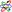## Separatrix

A phase curve (invariant Manifold) which meets a Hyperbolic Fixed Point (intersection of a stable and an unstable invariant Manifold). A separatrix marks a boundary between phase curves with different properties. For example, the separatrix in the equation of motion for the pendulumoccurs at the angular momentumwhere oscillation gives way to rotation.